## Data-Hack SQL注入检测

2015-03-12 10:02:00

• 收集数据
• 思考数据
• 特征工程
• 机器学习

# 0x01 准备

## 1. tools

sqlparse (一个用于解析sql语法树的库) Scikit-Learn (python机器学习库) Pandas (用于快速处理一定量的数据) numpy (用于科学计算) matplotlib (用于数据可视化)

# 0x02 准备数据

``````# -*- coding: utf-8 -*-
import os
import pandas as pd

basedir = '/Users/slay/project/python/datahack/data_hacking/sql_injection/data'
filelist = os.listdir(basedir)
df_list = []

# 循环读取 basedir下面的内容，文件名为 'legit'的是合法内容，malicious的是 恶意sql语句
for file in filelist:
df['type'] = 'legit' if file.split('.') == 'legit' else 'malicious'
df_list.append(df)

# 将内容放入 dataframe对象
dataframe = pd.concat(df_list, ignore_index=True)

dataframe.dropna(inplace=True)

# 统计内容
print dataframe['type'].value_counts()

# 查看前五个
``````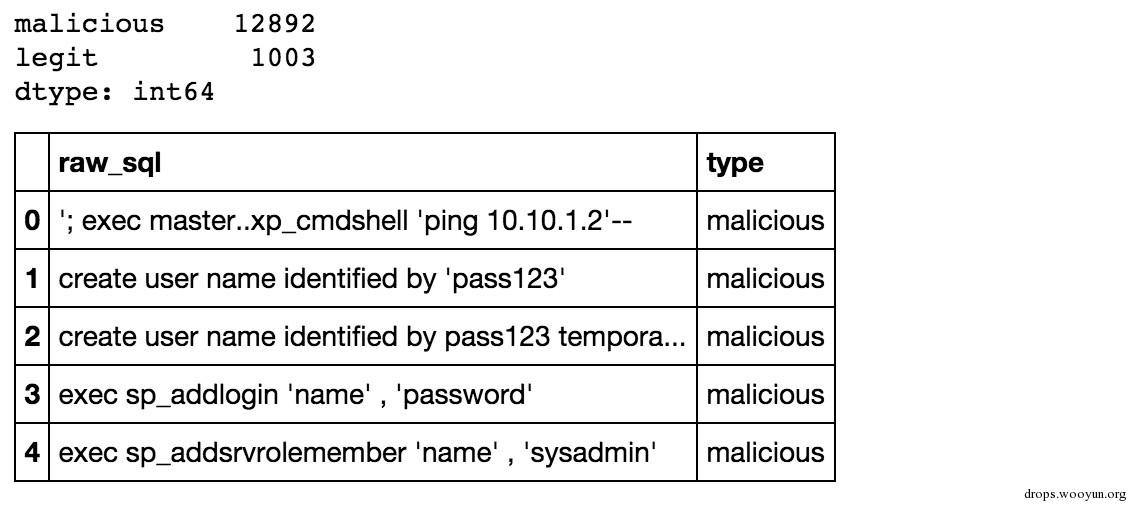# 0x03 特征工程

## 1. 概念

``````select user from admin;
``````

## 2. 转化数据

``````import sqlparse
import string

def parse_sql(raw_sql):
parsed_sql = []
sql = sqlparse.parse(unicode(raw_sql,'utf-8'))
for parse in sql:
for token in parse.tokens:
if token._get_repr_name() != 'Whitespace':
parsed_sql.append(token._get_repr_name())
return parsed_sql

sql_one = parse_sql("select 2 from admin")
sql_two = parse_sql("INSERT INTO Persons VALUES ('Gates', 'Bill', 'Xuanwumen 10', 'Beijing')")

print "sql one :%s"%(sql_one)
print "sql two :%s"%(sql_two)
``````

``````dataframe['parsed_sql'] = dataframe['raw_sql'].map(lambda x:parse_sql(x))
``````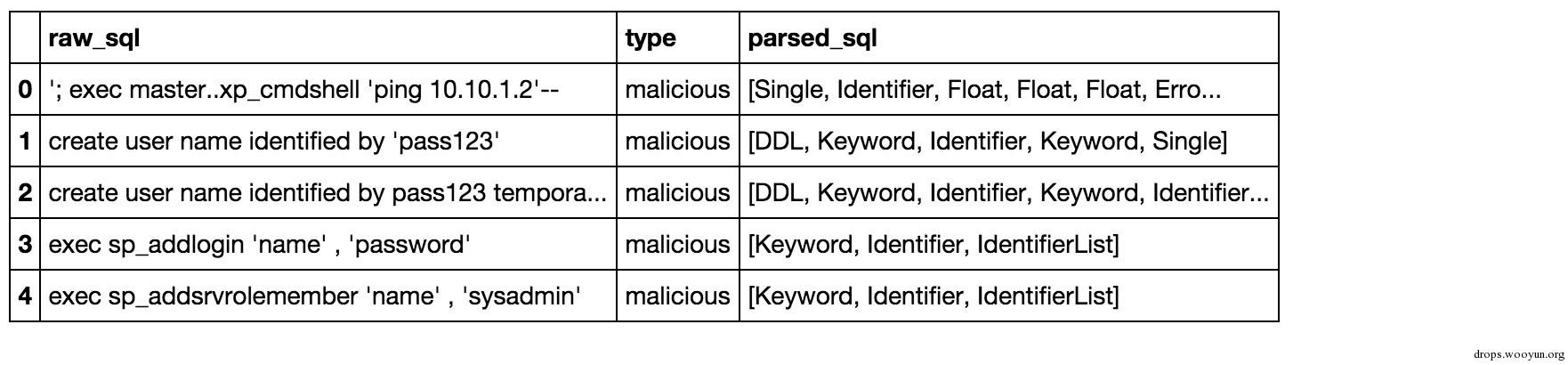## 3. Other

``````dataframe['len'] = dataframe['parsed_sql'].map(lambda x:len(x))
``````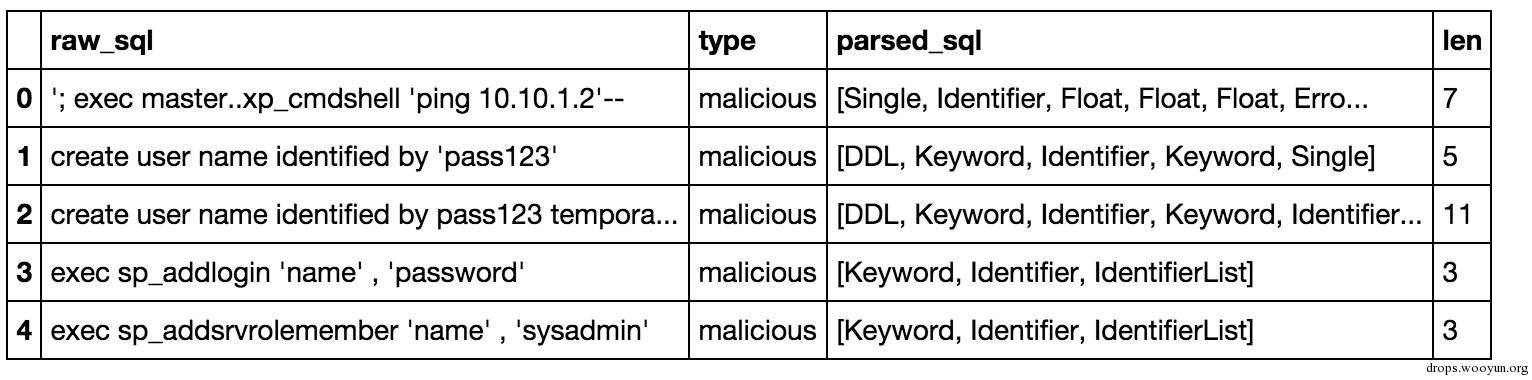``````%matplotlib inline
import matplotlib.pyplot as plt
dataframe.boxplot('len','type')
plt.ylabel('SQL Statement Length')
``````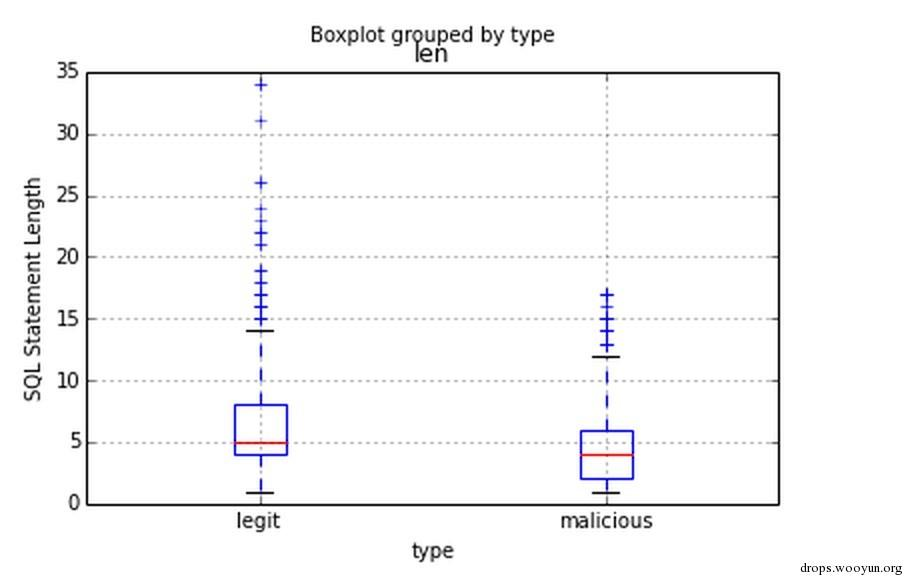# 0x04 机器学习

## 1. Train & Test

Gilles Louppe《随机森林：从理论到实践》 http://arxiv.org/pdf/1407.7502v1.pdf

``````import numpy as np
from sklearn.preprocessing import LabelEncoder
from sklearn.feature_extraction.text import CountVectorizer
import string

vectorizer = CountVectorizer(min_df=1)
le = LabelEncoder()

X = vectorizer.fit_transform(dataframe['parsed_sql'].map(lambda x:string.join(x,' ')))

x_len = dataframe.as_matrix(['len']).reshape(X.shape,1)

x = X.toarray()

y = le.fit_transform(dataframe['type'].tolist())

print x[:100]
print y[:100]
``````

``````[[0 0 0 ..., 2 0 0]
[0 0 0 ..., 1 0 0]
[0 0 0 ..., 0 0 0]
...,
[0 0 0 ..., 0 0 0]
[0 0 0 ..., 0 0 0]
[0 0 0 ..., 0 0 0]]
[1 1 1 1 1 1 1 1 0 0 0 0 0 0 0 0 0 0 0 0 0 0 0 0 0 0 0 0 0 0 0 0 0 0 0 0 0
0 0 0 0 0 0 0 0 0 0 0 0 0 0 0 0 0 0 0 0 0 0 0 0 0 0 0 0 0 0 0 0 0 0 0 0 0
0 0 0 0 0 0 0 0 0 0 0 0 0 0 0 0 0 0 0 0 0 0 0 0 0 0]
``````

``````clf = sklearn.ensemble.RandomForestClassifier(n_estimators=30)

scores = sklearn.cross_validation.cross_val_score(clf, x, y, cv=10, n_jobs=4)

print scores
``````

``````[ 0.97699497  0.99928109  0.99928058  1.          1.          0.97192225
0.99928006  0.99856012  1.          1.        ]
``````

``````from sklearn.cross_validation import train_test_split
# 将数据分割为 训练数据 和 测试数据，训练数据用于训练模型，测试数据用于测试分类器性能。
X_train, X_test, y_train, y_test, index_train, index_test = train_test_split(x, y, dataframe.index, test_size=0.2)
# 开始训练
clf.fit(X_train, y_train)
# 预测
X_pred = clf.predict(X_test)
``````

``````%matplotlib inline
import matplotlib.pyplot as plt
from sklearn.metrics import confusion_matrix

cm = confusion_matrix(X_pred,y_test)
print cm

# Show confusion matrix in a separate window
plt.matshow(cm)
plt.title('Confusion matrix')
plt.colorbar()
plt.ylabel('True label')
plt.xlabel('Predicted label')
plt.show()
``````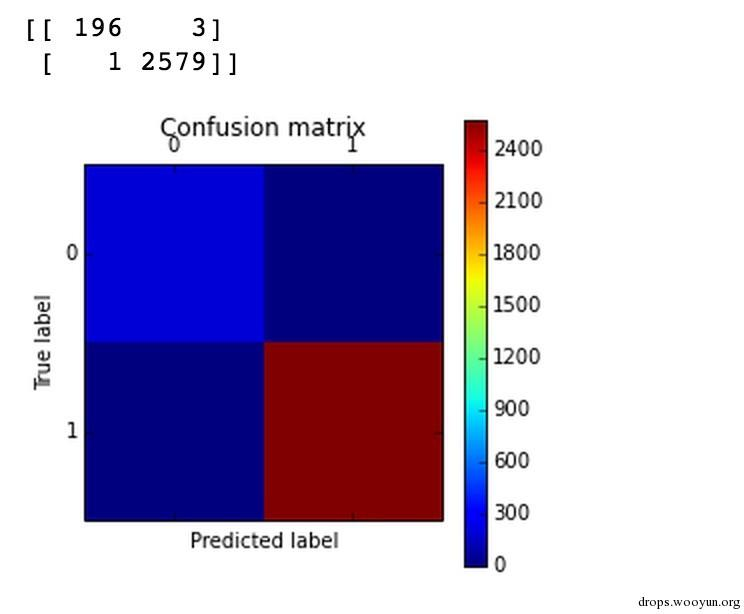## 2. 调整

sklearn大部分的模型有个功能叫predict_proba，就是说预测的概率，predict其实就是内部调用下predict_proba，然后按50%。我们可以装变一下直接调用predict_proba，让我们自己调整分类的概率。

``````loss = np.zeros(2)
y_probs = clf.predict_proba(X_test)[:,1]
thres = 0.7 # 用0.7的几率来分类
y_pro = np.zeros(y_probs.shape)
y_pro[y_probs>thres]=1.
cm = confusion_matrix(y_test, y_pro)
print cm
``````

``````[[ 197    0]
[   5 2577]]
``````

legit被错误分类的概率降低了，但是0.7只是我们随意想出来的一个参数，能不能简单的想个办法优化一下呢？让我们简单定义一个函数f(x),会随着我们输入的参数输出误分类的概率。

``````def f(s_x):
loss = np.zeros(2)
y_probs = clf.predict_proba(X_test)[:,1]
thres = s_x # This can be set to whatever you'd like
y_pro = np.zeros(y_probs.shape)
y_pro[y_probs>thres]=1.
cm = confusion_matrix(y_test, y_pro)
counts = sum(cm)
count = sum(counts)
if counts>0:
loss=float(cm[0,1])/count
else:
loss=0.01
if counts>0:
loss=float(cm[1,0])/count
else:
loss=0.01
return loss

# 0.1 到 0.9 之前的 100个数值
x = np.linspace(0.1,0.9,100)
# x输入f(x)之后得到的结果
y = np.array([f(i) for i in x])
# 可视化
plt.plot(x,y)
plt.show()
``````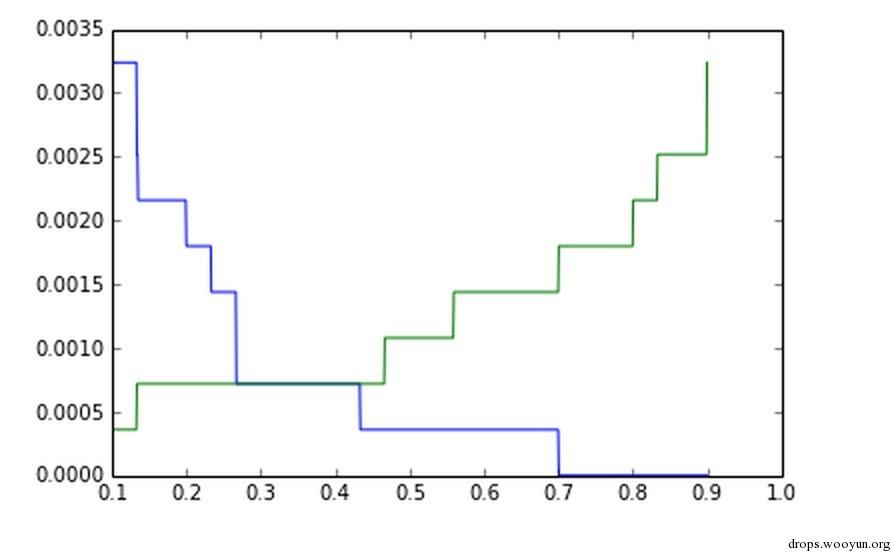# 0x05 结语

### 路

2015-03-12 10:11:07

### 我

2015-03-12 10:19:50

### 小

2015-03-12 10:29:36

### S

2015-03-12 14:55:12

### T

2015-03-12 15:59:39

### D

2015-03-12 16:36:502015-03-12 18:45:152015-03-12 21:56:26

### S

2015-03-13 09:44:42

### 麻

2015-03-14 12:05:50

### 寂

2015-03-15 12:36:59

### 路

2015-03-16 11:11:05

feifei女神说,RF没问题,但sqlparse解析粒度过粗,导致泛化误差过大

### 大

2015-03-16 12:23:38

### 大

2015-03-16 12:29:27

### 路

2015-03-16 12:38:10

feifei女神说,n-gram还不如sqlparse。。。

### 一

2015-03-17 13:43:39

feifei女神是谁0 0

### F

2015-03-22 21:20:37

### J

2015-03-25 10:50:20

### 路

2015-04-02 16:15:00

### 路

2015-04-02 16:16:53

### 大

#### 大学生### 随机分类

Windows安全 文章：88 篇

Python安全 文章：13 篇
XSS 文章：34 篇

### 扫码关注公众号### 你

#### 你又不是她#### gxh191

zgr！！！yyds